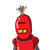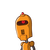# The daliy income of a vegatable vendor on six days was180,226,230,250,190,217what is the average of the daliy income​

The daliy income of a vegatable vendor on six days was180,226,230,250,190,217what is the average of the daliy income​

### 2 thoughts on “The daliy income of a vegatable vendor on six days was180,226,230,250,190,217what is the average of the daliy income​”

1.=180+226+230+250+190+217/2

=1293/2

=646.5

2.Average = total sum of all values / no. of values

average daily income

= 180+226+230+250+190+217 / 6

= 1293 / 6

= 215.5